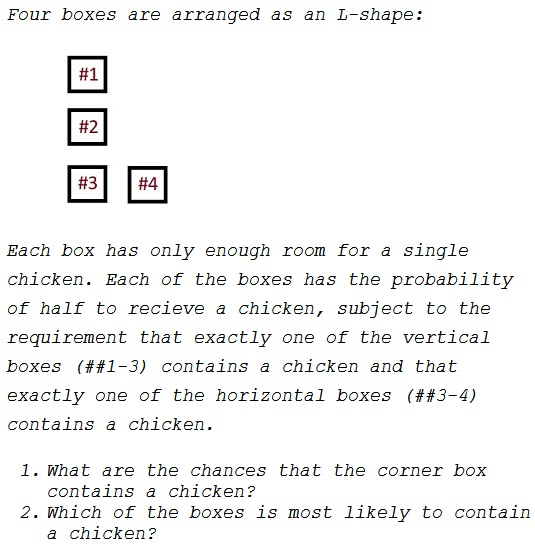# Chickens in Boxes

### Problem### Solution 1

All-in-all, there are $16$ equiprobable ways to place chickens into four boxes. Only three of them satisfy the conditions of the problem:

$\begin{array}{ccccc} \textit{Box #}&1&2&3&4\\ &\text{chicken}&&&\text{chicken}\\ &&\text{chicken}&&\text{chicken}\\ &&&\text{chicken}&& \end{array}$

Thinking of the three possibilities as equiprobable, the corner box (#3) contains a chicken only once; hence the chance of this happening is $1:2.$ The same is true of boxes #1 and #2. The box #4, on the other hand, contains a chicken twice, hence with the chances of $2:1.$

### Solution 2

Probability that the corner box contains a chicken, provided there's only one chicken, $P(B_3|\text{1 chicken})=1;$ in case of two chickens, $P(B_3|\text{2 chickens})=0.$ The first event occurs in one case (see the table above), the second event occurs in two cases. Therefore, the chances that the corner box contains a chicken are "1:2."

Similarly, for the Box #4, $P(B_4|\text{1 chicken})=0;$ $P(B_4|\text{2 chickens})=1.$ The first event occurs in one case, the second in two. Hence, for the Box #4, the chances are $2:1.$

By the same reasoning, $P(B_1|\text{1 chicken})=0;$ $P(B_1|\text{2 chickens})=\displaystyle \frac{1}{2}.$ So the chances, for $B_1$ (and, similarly, $B_2$) are $\displaystyle \frac{1}{2}:1=1:2.$

### Acknowledgment

The problem is from P. J. Nahin's Will You Be Alive 10 Years from Now? (Princeton University Press, 2013). Nahin attributes the problem to one of Merilyn von Savant's readers.

The above is an adaptation of Nahin's solution.